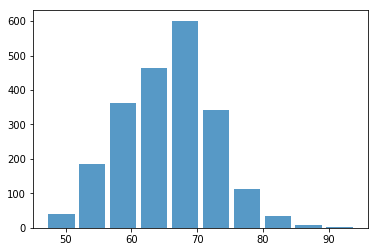# Step 4: Pre-process data

Workshop Resources

By now, we have imported our dataset. In real life, each soccer player has a specific position. Different positions require strength in different attributes. So let’s narrow down the scope to the striker.

First, let’s list all the positions. This statement looks a little bit longer, but it does the work. The fifa_data['position'] selects position column of the fifa_data, the dropna() eliminates cells that are blank, and unique() remove all duplicated items for us.

# to find out how many positions are there
print(fifa_data['Position'].dropna().unique())

['RF' 'ST' 'LW' 'GK' 'RCM' 'LF' 'RS' 'RCB' 'LCM' 'CB' 'LDM' 'CAM' 'CDM'
'LS' 'LCB' 'RM' 'LAM' 'LM' 'LB' 'RDM' 'RW' 'CM' 'RB' 'RAM' 'CF' 'RWB'
'LWB']


Now we can filter data by position “ST”. You’re encouraged to select other positions to see what’s the difference.

# get players by position
fifa_data_by_pos = fifa_data[fifa_data['Position']=='ST']


Let’s plot a histogram for overall ratings of all strikers.

plt.hist(x=fifa_data_by_pos[target], bins=10, alpha=0.75, rwidth=0.85)

(array([ 40., 186., 363., 463., 601., 341., 113.,  34.,   9.,   2.]),
array([47. , 51.7, 56.4, 61.1, 65.8, 70.5, 75.2, 79.9, 84.6, 89.3, 94. ]),
<a list of 10 Patch objects>)Next, we want to split the data into two sets, one is used to train the model, another one is used to verify the trained model is good.

You may think, we should leave as much as possible data for training because it makes the model better. The model fits better, but only for training datasets. When you apply the model to testing data, the prediction accuracy could go down. This is called “overfitting.”

Now, we leave 25% of the data for testing.

# split data into train_data and test_data randomly
# you're weclome to change the ratio of test_size to see what will happen
train_data, test_data = train_test_split(fifa_data_by_pos,test_size=0.25)

# print the number of players in train_data and test_data
# len() gives you the number of players in numerical format
# str() converts numerical value into string
print("The # of training data is " + str(len(train_data)))
print("The # of testing data is " + str(len(test_data)))

The # of training data is 1614
The # of testing data is 538